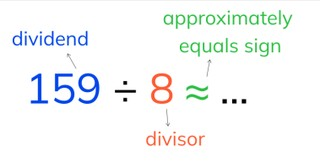Estimated division with numbers to 1,000 by a number less than 10

# Estimated division with numbers to 1,000 by a number less than 10

Students learn to estimate quotients to 1,000 divided by numbers <10.8,000 schools use Gynzy92,000 teachers use Gynzy1,600,000 students use Gynzy

## General

The students learn to estimate the quotient of a number to 1,000 divided by a number less than 10 by rounding the dividend to a number that is divisible by the divisor.

## Standards

CCSS.Math.Content.4.NBT.B.6

## Learning objective

Students will be able to estimate quotients with numbers to 1,000 divided by a number less than 10.

## Introduction

Together with the students, you practice solving division problems presented in three ways: with visual support, abstract, and in the form of a story problem.

## Instruction

First you explain what the dividend, the divisor, and the approximately equals sign is. Then you tell the students that with estimated division you always look for a dividend that is divisible by the divisor. Tell that estimated division is different than estimated addition or subtraction, where you can more easily round to rounder numbers. With estimated division you cannot use all round numbers. You show how estimating division problems works step by step. Explain that you first look at the numbers in the table of the divisor. Find which outcome is the closest to the dividend. After that you can solve the problem. Next the students practice finding the division problem that is closest to the estimated division problem. After this, the students are going to estimate division problems on their own. For this, explain that it is sometimes possible to round the dividend to a tens- or hundreds number. Show this step by step through the problem 323 ÷ 4 ≈. Then have the students estimate the following problems on their own and ask how they rounded the dividend. After this, you discuss as a group whether the answers are more or less than the estimate and how you can know that. Then walk through the steps of solving an estimated division problem in a story problem

Check whether the students can estimate division problems by asking them the following questions:
- When is it useful to estimate quotients?
- What do you need to pay attention to when estimating division problems?

## Quiz

The students test their understanding of estimated division with a number to 1,000 by a number less than 10 through ten exercises. In each of these exercises, the students must estimate the quotient. Some of the exercises are story problems.

## Closing

You discuss once again with the students that it is important to be able to estimate division problems, because then you can quickly know approximately how much something can be divided by. Check if the students have understood the instruction section by having them answer a yes or no question. If the answer is "yes," then they put their arms up, and if the answer is "no," they put their arms down. Have the students explain why they answered the way they did.

## Teaching tips

When students have difficulty with estimating division problems with numbers to 1,000 by a number less than 10, you can first have them practice with smaller division problems that don't have numbers greater than 200. You can also make a visual aid using blocks. Next you make division problems with increasingly larger numbers. Habe them write down the rounded numbers that are divisible by the divisor to choose the correct estimated quotient.

### The online teaching platform for interactive whiteboards and displays in schools

• Save time building lessons

• Manage the classroom more efficiently

• Increase student engagement